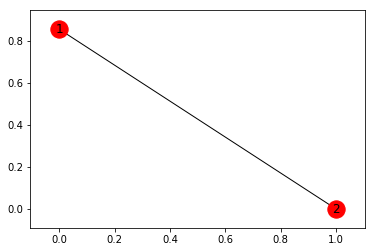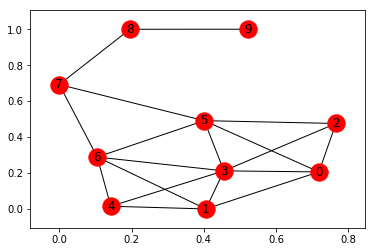## 我的说说## 导航标题## 自己想吧# 使用python画社交网络图实例代码

piaodoo 编程教程 2020-02-02 11:42:02 65 0 python教程

```import networkx as nx
import matplotlib.pyplot as plt
G = nx.Graph()
nx.draw_networkx(G)
plt.show``````import networkx as nx
import matplotlib.pyplot as plt
G = nx.krackhardt_kite_graph()
nx.draw_networkx(G)
plt.show```阿尔贝托·博斯凯蒂, 卢卡·马萨罗(作者),于俊伟,靳小波(译者)., 数据科学导论：Python语言实现 (北京: 机械工业出版社, 2016).

 Tony Ojeda(托尼·奥杰德)., 数据科学实战手册(R+Python) (北京: 人民邮电出版社, 2016).  (书中代码及数据)

 拉塞尔 (Matthew A.Russell)(作者)., 社交网站的数据挖掘与分析 (北京: 机械工业出版社, 2015). (书中代码)